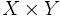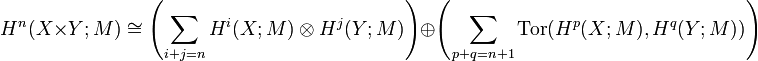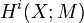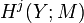# Kunneth formula for cohomology

## Statement

Suppose$X$ and$Y$ are topological spaces. We then have the following relation betwen the cohomology groups of$X$,$Y$, and the product space$X \times Y$.

For any$n \ge 0$ and any module$M$ over a principal ideal domain$R$, we have:$\! H^n(X \times Y; M) \cong \left(\sum_{i + j = n} H^i(X;M) \otimes H^j(Y;M)\right) \oplus \left(\sum_{p + q = n + 1} \operatorname{Tor}(H^p(X;M),H^q(Y;M))\right)$

Here,$\operatorname{Tor}$ is torsion of modules over the ring$R$.

## Particular cases

### Case of free modules

If all the cohomology groups$H^i(X;M)$ are free (or more generally, torsion-free) modules over$R$, and/or all the cohomology groups$H^j(Y;M)$ are free (or more generally, torsion-free) modules over$R$, then all the torsion part vanishes and we simply get:$\! H^n(X \times Y; M) \cong \left(\sum_{i + j = n} H^i(X;M) \otimes H^j(Y;M)\right)$